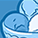# The meaning of position2go 'i_mean', 'if_scale', and 'fft_window'Level 1
Level 1
Hello, I have a question while interpreting position2go in Korea.
Content may be strange using a translator

complex_fft_signal [2 * idx + 0] = (float) (i_data [idx]-* i_mean) * if_scale * fft_window [idx * dec_idx]; I am not sure what that means when interpreting this code.

1. The reason for subtracting 'i_mean' (the reason for subtracting the average value)
2. Meaning of 'if_scale'(why multiply if_scale)
3. Advantages of using 'fft_window'(window function convolution??)

thank you
0 Replies